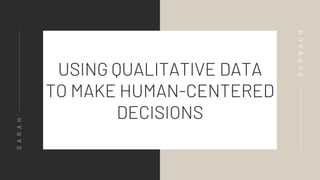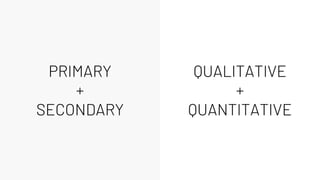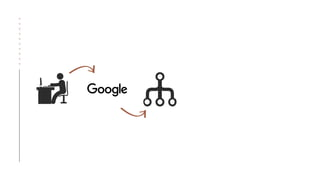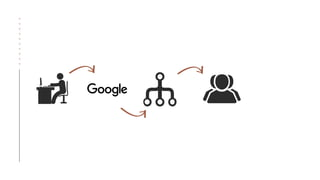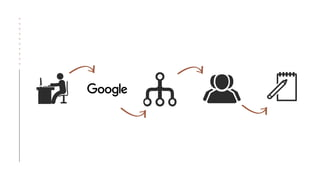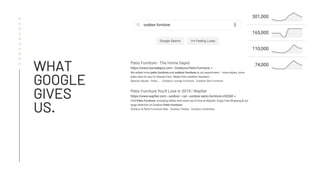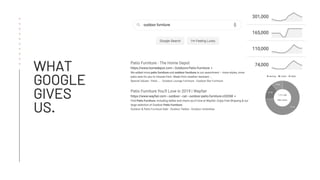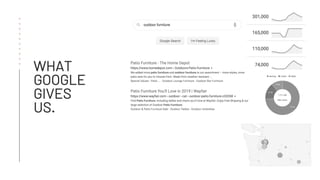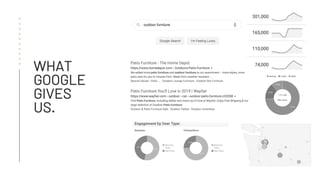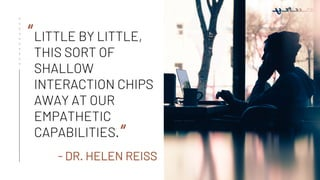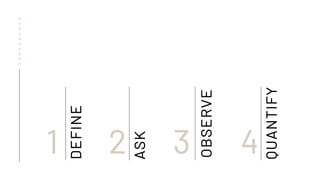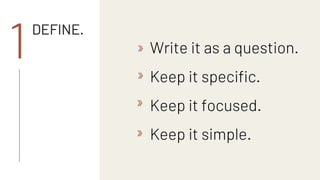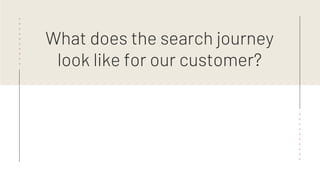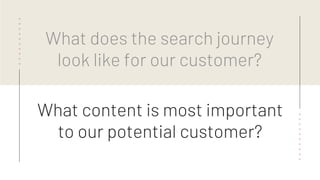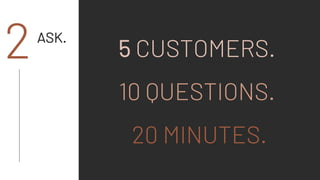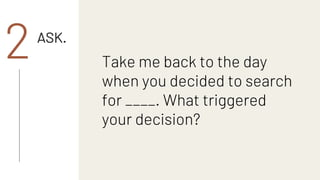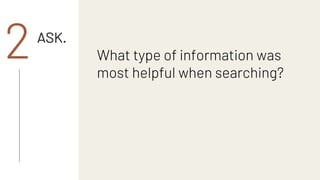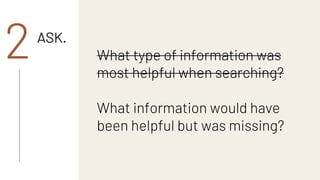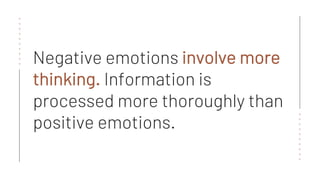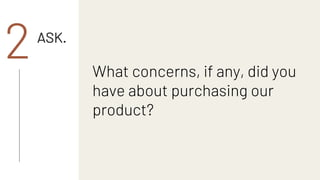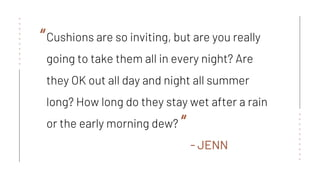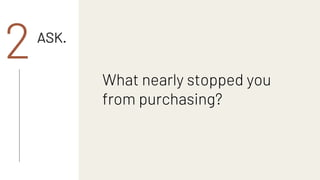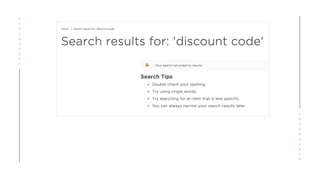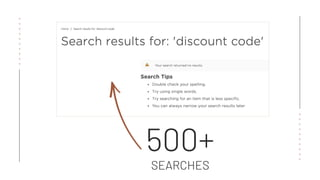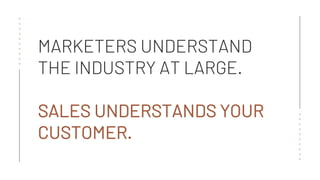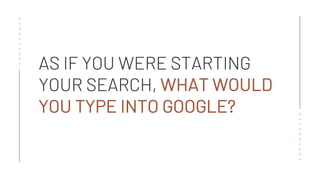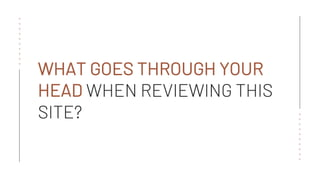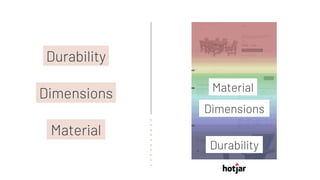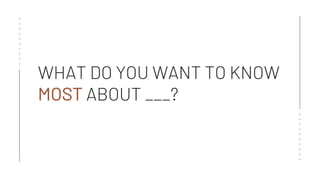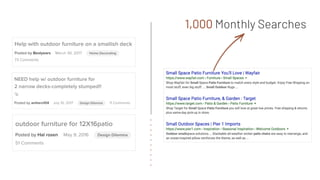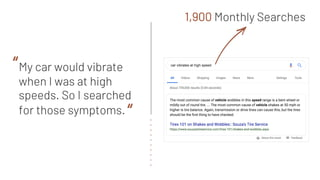1 of 68

### SearchLove London 2019 - Sarah Gurbach - Using Qualitative Data to Make Human-centered Decisions

1. USING QUALITATIVE DATA TO MAKE HUMAN-CENTERED DECISIONS SARAH GURBACH
2. PRIMARY + SECONDARY
3. PRIMARY + SECONDARY QUALITATIVE + QUANTITATIVE
4. THE RATIONAL WORLD OF ALGORITHMS IS NOT A REFLECTION OF HOW HUMANS MAKE DECISIONS. <<<<<<<<<<
5. <<<<<<<<<<
6. <<<<<<<<<<
7. <<<<<<<<<<
8. <<<<<<<<<<
9. <<<<<<<<<<
10. Data are people, too.
11. WHAT GOOGLE GIVES US. <<<<<<<<<<
12. WHAT GOOGLE GIVES US. <<<<<<<<<<
13. WHAT GOOGLE GIVES US. <<<<<<<<<<
14. WHAT GOOGLE GIVES US. <<<<<<<<<<
15. WHAT GOOGLE GIVES US. <<<<<<<<<<
16. WHAT GOOGLE GIVES US. <<<<<<<<<<
17. WHAT GOOGLE GIVES US. <<<<<<<<<<
18. LITTLE BY LITTLE, THIS SORT OF SHALLOW INTERACTION CHIPS AWAY AT OUR EMPATHETIC CAPABILITIES. <<<<<<<<<< “ “ - DR. HELEN REISS
19. WHAT OUR CUSTOMER GIVES US. <<<<<<<<<<
20. WHAT OUR CUSTOMER GIVES US. <<<<<<<<<<
21. WHAT OUR CUSTOMER GIVES US. <<<<<<<<<<
22. WHAT OUR CUSTOMER GIVES US. <<<<<<<<<<
23. WHAT OUR CUSTOMER GIVES US. <<<<<<<<<<
24. WHAT OUR CUSTOMER GIVES US. <<<<<<<<<<
25. WHAT OUR CUSTOMER GIVES US. <<<<<<<<<<
26. WHAT OUR CUSTOMER GIVES US. <<<<<<<<<< NEEDS WANTS BARRIERS TENDENCIES VALUES BIASES
27. MINIMAL_ <<<<<<<<<< <<<<<<<<<<
28. DEFINE 1 <<<<<<<<<< ASK 2 OBSERVE 3 QUANTIFY 4
29. DEFINE. 1 Write it as a question. Keep it specific. Keep it focused. Keep it simple.
30. MINIMAL_ <<<<<<<<<< <<<<<<<<<< What does the search journey look like for our customer?
31. MINIMAL_ <<<<<<<<<< <<<<<<<<<< What does the search journey look like for our customer? What content is most important to our potential customer?
32. THERE IS NO SUBSTITUTE FOR TALKING TO YOUR CUSTOMER.
33. ASK. 2 5 CUSTOMERS. 10 QUESTIONS. 20 MINUTES.
34. ASK. 2 Take me back to the day when you decided to search for ____. What triggered your decision?
35. STORIES ARE AUTHENTIC HUMAN EXPERIENCES. THEY CONNECT US.
36. ASK. 2 What type of information was most helpful when searching?
37. ASK. 2 What type of information was most helpful when searching? What information would have been helpful but was missing?
38. <<<<<<<<<< <<<<<<<<<< Negative emotions involve more thinking. Information is processed more thoroughly than positive emotions.
40. <<<<<<<<<< Cushions are so inviting, but are you really going to take them all in every night? Are they OK out all day and night all summer long? How long do they stay wet after a rain or the early morning dew? - JENN <<<<<<<<<< “ “
42. <<<<<<<<<< <<<<<<<<<<
43. <<<<<<<<<< <<<<<<<<<< 500+ SEARCHES
44. <<<<<<<<<< <<<<<<<<<< MARKETERS UNDERSTAND THE INDUSTRY AT LARGE. SALES UNDERSTANDS YOUR CUSTOMER.
45. OBSERVE. 3
46. <<<<<<<<<< <<<<<<<<<< AS IF YOU WERE STARTING YOUR SEARCH, WHAT WOULD YOU TYPE INTO GOOGLE?
47. <<<<<<<<<< <<<<<<<<<< WHAT GOES THROUGH YOUR HEAD WHEN REVIEWING THIS SITE?
48. IT’S HUMBLING TO WATCH YOUR CUSTOMER STRUGGLE BECAUSE OF SOMETHING YOU DID.
49. What are you looking for that you cannot find?
50. <<<<<<<<<< Durability Dimensions Material
51. <<<<<<<<<< Durability Dimensions Material Material Dimensions Durability
52. THE ONE QUESTION SURVEY
53. <<<<<<<<<< <<<<<<<<<< WHAT DO YOU WANT TO KNOW MOST ABOUT ___?
54. CLOSE THE GAP BETWEEN WHAT YOU THINK THEY WANT AND WHAT THEY REALLY WANT.
55. bit.ly/qualt-love
56. QUANTIFY. 4DECISION
57. <<<<<<<<<< 1,000 Monthly Searches
58. <<<<<<<<<< My car would vibrate when I was at high speeds. So I searched for those symptoms. “ “ 1,900 Monthly Searches
59. Big data can tell you what people do at scale, but only your customer can tell you what they’re thinking and why they do what they do. <<<<<<<<<<
60. JUST DON’T FORGET WHO WE’RE DOING THIS FOR. <<<<<<<<<<
61. <<<<<<<<<< <<<<<<<<<< THANK YOU